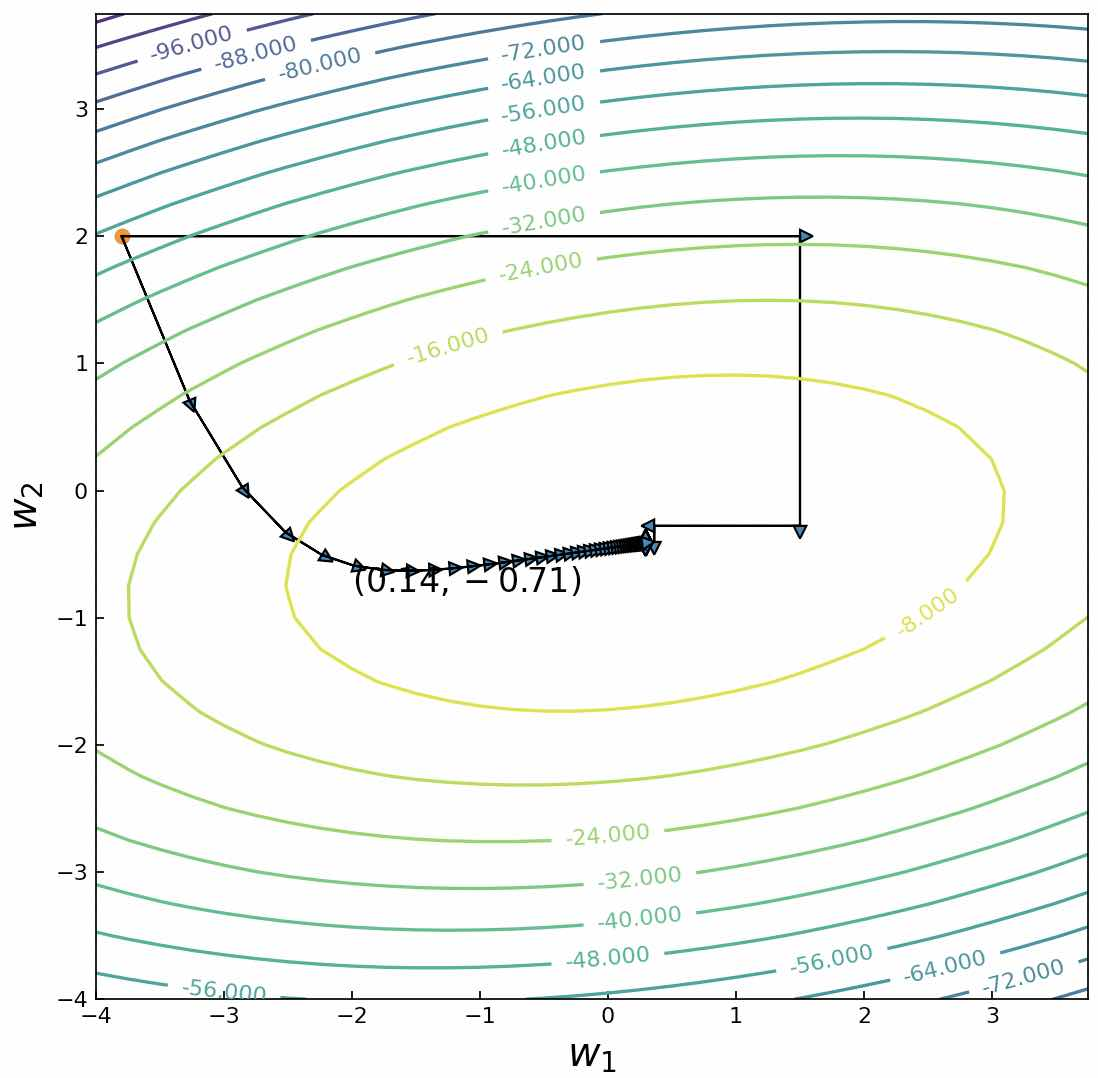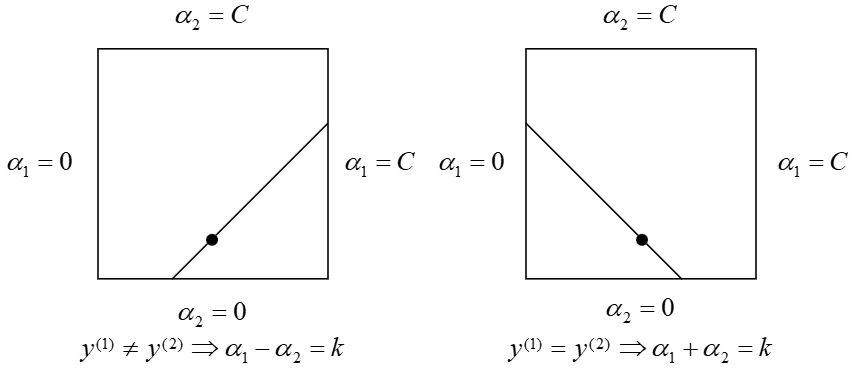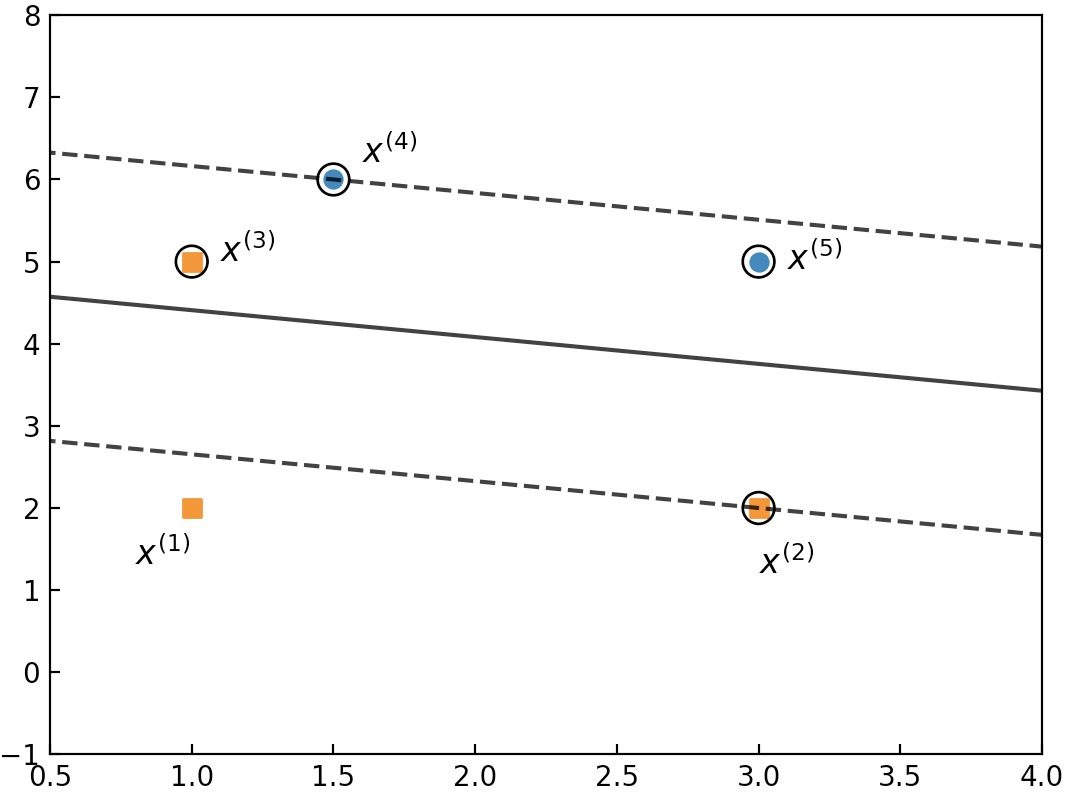### 2 SVM软间隔求解

#### 2.1 坐标上升算法(1) 随机初始化向量$w$为初始参数值；

(2) 在${{w}_{1}},{{w}_{2}},...,{{w}_{n}}$中依次选择${{w}_{i}},i=1,2,...,n$为变量固定其它参数为常量，然后求目标函数关于${{w}_{i}}$的导数并令其为0求得${{w}_{i}}$

(3) 重复执行步骤(2)直到目标函数收敛或者是误差小于某一阈值结束。

#### 2.3 SMO算法原理

${{\alpha }_{1}}=(\zeta -{{\alpha }_{2}}{{y}^{(2)}}){{y}^{(1)}}$代入式$(13)$便可以得到一个只含有变量${{\alpha }_{2}}$目标函数#### 2.5 SVM算法求解示例

xxxxxxxxxx71$C$惩罚项系数；2​3$tol$：误差容忍度；4​5$max\_passes$：当$\alpha_i$不再发生变化时继续迭代更新的最大次数；6​7$(({{x}^{(1)}},{{y}^{(1)}}),...,({{x}^{(m)}},{{y}^{(m)}}))$：训练集；

xxxxxxxxxx31$\alpha\in\mathbb{R}^m$：求解得到的拉格朗日乘子；2​3$b\in\mathbb{R}$：求解得到的偏置。
xxxxxxxxxx251初始化所有 alpha_i = 0, b = 0, passes = 02while (passes < max_passes)3    num_changed_alphas = 04    for i = 1,...,m5        计算 E_i6        if ((y_i*E_i < -tol and a_i < C)||(y_i*E_i > tol and alpha_i > 0))7            随机选择j，且j不等于i8            计算 E_j9            保存：alpha_i_old = alpha_i,alpha_j_old = alpha_j10            计算 L 和 H11            if (L == H):12                continue13            计算 eta14            if (eta >- 0):15                continue16            计算 alpha_j并裁剪17            if (|alpha_j - alpha_j_old| < 10e-5):18                continue19            分别计算alpha_i, b_1, b_220            计算b21            num_changed_alphas += 122    if (num_changed_alphas == 0):23        passes += 124    else:25        passes = 0

xxxxxxxxxx71data_x = np.array([[5, 1], [0, 2], [1, 5], [3., 2], [1, 2], [3, 5], [1.5, 6], [4.5, 6], [0, 7]])2data_y = np.array([1, 1, 1, 1, 1, -1, -1, -1, -1])3alphas, b = smo(C=.2, tol=0.001, max_passes=200, data_x=data_x, data_y=data_y)4print(alphas) #[0.   0.   0.2   0.142   0.   0.2   0.142   0.   0.]5print(b)# 2.666w = compute_w(data_x,data_y,alphas)7print(w)# [-0.186,  -0.569]### 引用

 John C. Platt. Sequential Minimal Optimization: A Fast Algorithm for Training Support Vector Machines, Microsoft Research Technical Report MSR-TR-98-14.

 Andrew Ng, Machine Learning, Stanford University, CS229, Spring 2019.

 Machine Learning, Stanford University, CS229, Autumn 2009.